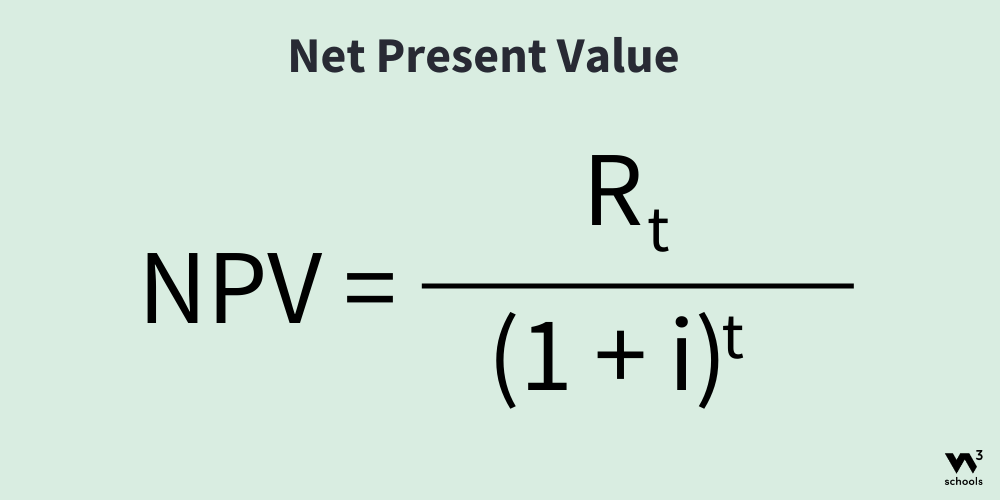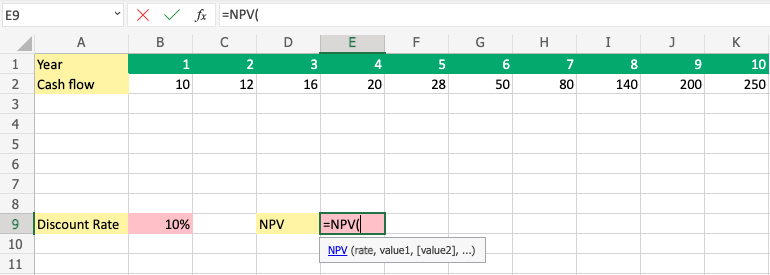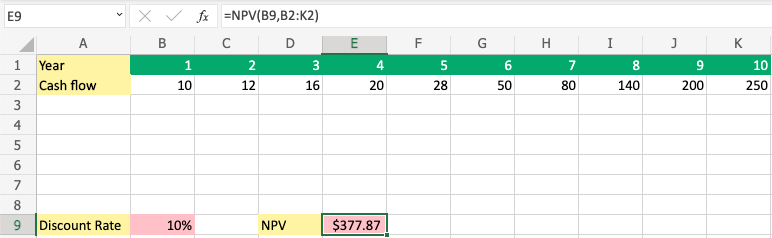# How to Calculate NPV Using Excel

Learn how to calculate NPV (Net Present Value) using Excel.

NPV (Net Present Value) is a financial formula used to discount future cash flows.

The calculation is performed to find out whether an investment is positive in the future.

Keep in mind that money is always worth more today than in the future.

That is why we discount the future cash flows.

What else could you do with the money if not spent on this project?

## The NPV Formula

The Formula:• `R` = Return which defines the earnings, how much you have made.
• `t` = Time which used to define the duration of what you are calculating.
• `I` = Discount Rate which used to define your requirement of return.

## How to Calculate NPV Using Excel

##### Step 1) Create a sheet and set up values:

In this example, we will calculate the NPV over a 10 years period.

The Discount Rate, return of requirement is set to 10%.

Copy the values to follow the example.

Paste the values directly into your sheet.

You need to apply the styling on your own, if you want it to be more presentable.Copy Values

Ensure that you have `10%` (percentage) as input and not just `10` in cell `B9`##### Step 2) Start the NPV Function:
1. Select cell `E9`
2. Type `=NPV`
3. Select the `=NPV` function by clicking it##### Step 3) Enter NPV Values:
1. Select `B9` to Apply "rate"
2. Type `, or ;` to separate and move on to value
3. Select range `B2:K2`
4. Press the enter button

Note: The different parts of the function are separated by a symbol, like comma `,` or semicolon `;`

The symbol depends on your Language Settings.Nicely done!

You have successfully calculated the NPV for a 10 years period and applied a 10% Discount Rate.

In this case, the right answer was `377,87`W3Schools is optimized for learning and training. Examples might be simplified to improve reading and learning. Tutorials, references, and examples are constantly reviewed to avoid errors, but we cannot warrant full correctness of all content. While using W3Schools, you agree to have read and accepted our terms of use, cookie and privacy policy.